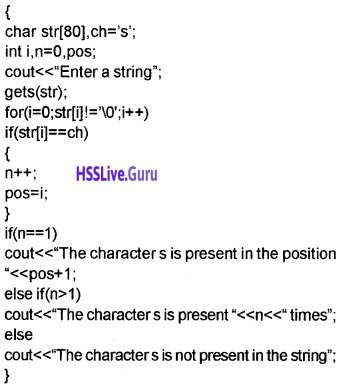# Plus Two Computer Application Chapter Wise Questions and Answers Chapter 2 Arrays

Students can Download Chapter 2 Arrays Questions and Answers, Plus Two Computer Application Chapter Wise Questions and Answers helps you to revise the complete Kerala State Syllabus and score more marks in your examinations.

## Kerala Plus Two Computer Application Chapter Wise Questions and Answers Chapter 2 Arrays

### Plus Two Computer Application Arrays One Mark Questions and Answers

Question 1.
From the following which is not true for an array
(a) It is easy to represent and manipulate array variable
(b) Array uses a compact memory structure
(c) Readability of program will be increased
(d) Array elements are dissimilar elements
(d) Array elements are dissimilar elements.

Question 2.
Consider the following declaration. int mark (50).
Is it valid? If no give the correct declaration.
It is not valid. The correct declaration is as follows, int mark. Use square brackets instead of parenthesis.Question 3.
Consider the following declaration. int mark.
The index of the last element is____.
199.

Question 4.
Consider the following declaration int mark
The index of the first element is_____
0.

Question 5.
Consider the following int age={15, 16, 17, 18};
From the following which type of initialisation is this.
(a) direct assignment
(b) along with variable declaration
(c) multiple assignment
(d) None of these
(b) along with variable declaration

Question 6.
From the following which is used to read and display array elements
(а) loops
(b) if
(c) switch
(a) loopsQuestion 7.
Write down the corresponding memory consumption in bytes

1. int age=_____
2. charname =_____
3. intage=____

1. 4*10 = 40 bytes (4 bytes for one integer)
2. 1*10=10 (one byte for each character)
3. 4*10*10 = 400 (4 * 100 elements)

Question 8.
Consider the following intage = {12, 13, 14};
cout<<age; What will be the output?
(а) 14
(b) 12
(c) 13
(d) 0
(d) 0

Question 9.
The elements of 2-dimensional array can be read using_____loop
nested loop.

Question 10.
_____is the process of reading / visiting elements of an array
traversal.

Question 11.
Anjaly wants to read the 10 marks that already stored in an array and find the total. This process is known as_____
(a) insertion
(b) deletion
(c) traversal
(d) linear search
(c) traversalQuestion 12.
The elements of an array of size ten are numbered from____to___.
0 to 9.

Question 13.
Element mark is which element of the array?
(a) The sixth
(b) the seventh
(c) the eighth
(d) impossible to tell
(b) the seventh

Question 14.
When a multidimensional array is accessed, each array index is
(a) Separated by column.
(b) Surrounded by brackets and separated by commas.
(c) Separated by commas and surrounded by brackets.
(d) Surrounded by brackets.
(d) surrounded by brackets

Question 15.
Write a C++ statement that defines a string variable called ‘name’ that can hold a string of upto 20 characters.
char name;

Question 16.
_____is a collection of elements with same data type
ArrayQuestion 17.
Following are some of the statements regarding array. Identify the correct statement.
(a) Array is a collection of elements of same data type.
(b) Array cannot be initialised during the time of declaration.
(c) Array allocates continuous memory.
(d) An array element can be accessed using index or subscript.
(a) Array is a collection of elements of same data type.

Question 18.
Which of the following is the correct declaration of an array?
(a) int a(10);
(b) int 10[a];
(c) a int;
(d) inta;
(d) int a;

Question 19.
Which is the last subscript of the array int m?
(a) 24
(b) 25
(c) 0
(d) 26
(a) 24

Question 20.
The memory size of the data type float is 4 bytes. What is the total bytes required for the array declaration float salary;?
(a) 10
(b) 4
(c) 40
(d) 400
(c) 4*10 = 40.Question 21.
int num; The above statement declares an array named num that can store maximum_____integer numbers.
(a) 99
(b) 100
(c) 101
(d) Any number
(b) 100

Question 22.
If int a; is array, then which element of the array will be referenced as a.
Fifth element.

Question 23.
Consider the following array declaration int A[ ] = {4, 5, 8}; int B[ ]={2, 10};
Write a valid C++ statement for finding the difference between the last element of the array ‘B’ and the first element of the array ‘A’.
B – A; ORA – B;

Question 24.
Consider the following code and predict the output.
int sum=0;
int a = {1, 2, 3, 4, 5};
for(i=0;i<4;++i)
{
sum=sum+a[i];
}
cout<<sum;
10.Question 25.
Which data type is used to declare a variable to hold string data?
The data type char is used for this.

Question 26.
The terminating character of string array is______
\0 or NULL character.

Question 27.
Write a statement for storing the string “NO SMOKING” using a character array with name ‘ARR’
of minimum size.
char APR = ”NO SMOKING”;

### Plus Two Computer Application Arrays Two Mark Questions and Answers

Question 1.
Given some array declaration. Pick the odd man out.
Float a[+40], int num[0-10], double . char name, amount of float.
char name. It is a valid array decalaration the remaining are not valid.

Question 2.
Whether the statement char text[] = “COMPUTER”; is True / False ? Justify.
It is a single-dimensional array. If the user doesn’t specify the size the operating system allocates the number of characters + one (for null character for text) bytes of memory. So here OS allocates 9 bytes of memory.Question 3.
Suppose you are given Total mark of 50 students in a class

1. How will you store these values using ordinary variable?
2. Is there any other efficient way to store these values? Give reason

We have to declare 50 variables individually to store total marks of 50 students. It is a laborious work. Hence we use array, it is an efficient way to declare 50 variables.

With a single variable name we can store multiple elements. Eg: int mark. Here we can store 50 marks. The first mark is in mark, second is in mark, …etc the fiftieth mark is in mark.

Question 4.
Consider the statement charstr[ ] = “PROGRAM” What will be stored in last location of this array. Justify
The last location is the null character(\0) because each string must be appedend by a null character.

Question 5.
Explain the needs for arrays
Array is collection of same type of elements. With the same name we can store more elements. The elements are distinguished by using its index or subscript. To store 50 marks of 50 students we have to declare 50 variables, it is a laborious work.

Hence the need for arrays arise. By using array this is very easy as follows int mark. Here the index of first element is 0, then 1, 2, 3, etc upto 49.Question 6.
Consider the following code and predict the output.
int a= {6, 8, 10,20,40};
cout<<“\n”<<a;
cout<<“\n”<<a+1;
20(The fourth element).
48(Sum of second element 8 and fifth element 40, i.e 8 + 40 =48).

Question 7.
Suppose you need to store the value 10, 20, 30, 40 and 50 into an array. Write different methods to do this problem. Answer:
Method 1:
int a={10, 20, 30, 40, 50};

OR

int a[ ]={10, 20, 30, 40, 50};

Method 2:
int a;
a=10;
a=20;
a=30;
a=40;
a=50;Question 8.
What would be the appropriate array declaration to store the following?

1. Name of a student
2. Age of 20 students
3. Mark of 6 subject
4. Average mark of 10 students in 5 subjects

1. charname;
2. intage;
3. intmark;
4. float mark;

Question 9.
Consider the following code and predict the output.
int A = {11, 12, 13, 14, 15};
int i;
for (i=4;i>=0;–i)
{
cout<<“\n”<<A[i];
}
It prints the array in reverse order as follows.
15
14
13
12
11.

Question 10.
Predict the output of the following code segment.
int K[ ] = {1, 2, 3, 4};
for (int i=0; i<4; i++)
cout<<K[i] * K[i]<<“\t”;
The output is as follows
1 4 9 16
Hint: 1(1*1) 4(2*2) 9(3*3) 16(4*4).Question 11.
Consider the following code and predict the output.
int A = {1, 2, 3, 4, 5, 6, 7, 8, 9, 10};
int sum=0, i;
for(i=0;i<10;++i)
{
if (A[i]%2==0)
{
sum=sum+A[i];
}
}
cout<<“\nSum=”<<sum;
The output is 30. That is sum of all even numbers in the array.

Question 12.
Predict the output of the following C++ statement.
char str = “WELCOME”;
cout<<“\n’’<,str;
cout<<“\n”<<str;
The output is as follows
C(The fourth character)
WELCOME.Question 13.
How many bytes will be allocated in the memory for storing the string “MY SCHOOL”? Justify your answer.
A total of 10 bytes. 9 bytes is used to store 9 characters in the string MY SCHOOL(including 1 byte for space) and 1 byte is used to store the NULL character.

### Plus Two Computer Application Arrays Three Mark Questions and Answers

Question 1.
Total mark of 50 students in a class are given in an array. A bonus of 10 marks is awarded to all of them. Write the program code for making such a modification.
# include
using namespace std;
int main()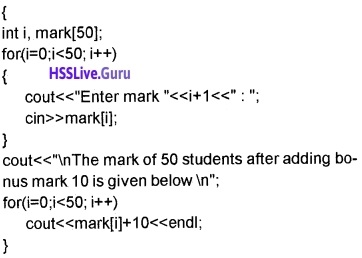Question 2.
Write the program code for counting the number of vowels from your school name
# include
# include
# include
using namespace std;
int main()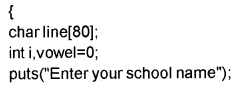Question 3.
Write the program code for counting the number of words from the given string.” Directorate of Higher Secondary Examination”
# include
# include
using namespace std;
int main()OR

(Program for more than one space between words)
# include
# include
# include
using namespace std;
int main()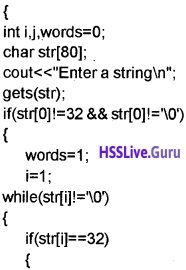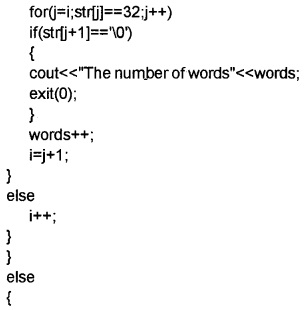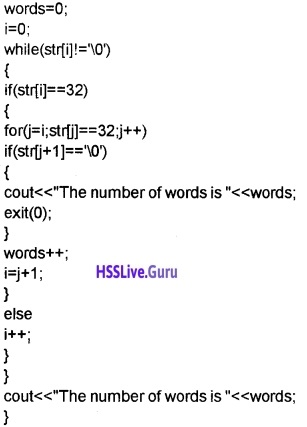Question 4.
Given a word like “ECNALUBMA” Write the program code for arranging it in into a meaningful word Answer:
# include
# include
# include
using namespace std;
int main()Question 5.
Explain different types of arrays.
1. Single dimensional:
It contains only one index or subscript. The index starts from 0 and ends with size-1.
Eg. int n; charname;

2. Multidimensional:
It contains more than one index or subscript. The two dimensional array contains two indices, one for rows and another for columns. The row index starts from 0 and end at row size-1 and column index starts at 0 and ends at colunn size-1.
Eg. int n can store 10 * 10 =100 elements. The index of the first element is n and index of the 100th element is n.Question 6.
Write a program to read the 5 marks of a students and display the marks and total. Answer:
# include
using namespace std;
int main()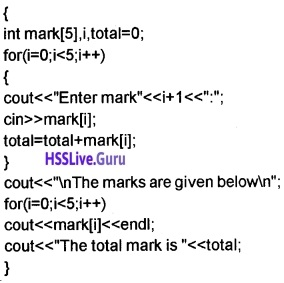Question 7.
Explain different array operations in detail.

1. Traversal:- All the elements of an array is visited and processed is called traversal
2. Search:- Check whether the given element is present or not
3. Sorting:-Arranging elements in an order is called sorting.

Question 8.
Given a word “COMPUTER”, write a C++ program to reverse the word without using any string functions.
# include
using namespace std;
int main()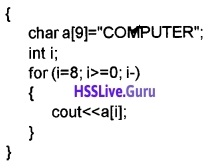Question 9.
Write statements to declare an array and initialize it with the numbers 1, 2, 3, 4, 5 and print 5, 4, 3, 2, 1.
# include
using namespace std;
int main()
int a={1, 2, 3, 4, 5},i;
for(i=4;i>=0;i-)
cout<<a[i]<<“,”;
}

Question 10.
Consider the following array declaration. Write statements to count how many numbers are greater than zero.
int p[ ] = {-5, 6, -7, 0, 8, 9};
# include
using namespace std;
int main()Question 11.
Write a C++ program to read 10 integer values and find the largest number among them using array.
# include
using namespace std;
int main()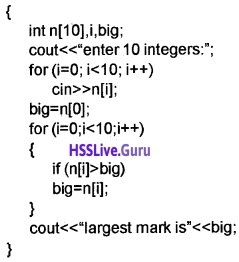Question 12.
Write a C++ program to accept a string from the keyboard and find its length without using function. For example if “WELCOME” is accepted, the output will be 7.
# include
# include
# include
using namespace std;
int main()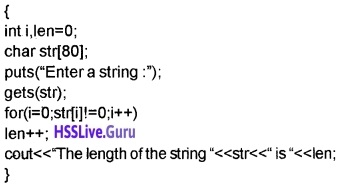Question 13.
Considerthe following C++ statements

1. charword;
cin>>word;
cout<<word;
2. char word;
gets(word);
puts(word);

If the string entered is “HAPPY NEW YEAR”. Predict the output in both cases and justify your answers.

1. HAPPY. When we use cin to accept string then space is the delimiter. The string after space is truncated.
2. HAPPY NEW YEAR. gets() reads all the characters (including space) upto the user presses the enter key.

Question 14.
Consider the following C++ statements
char str[] = “NO/nSMOKING”;
cout<<str;

1. What is the output of the above code?
2. How many bytes will be allocated in the memory for the variable str?

1. NO
SMOKING. The output is in 2 lines.
2. A total of 11 bytes is used to store this string.

1 byte for \n. 1 byte for \0(The null character that is automatically appended) and 9 bytes for the remaining characters (N, 0, S, M, 0, K, l, N AND G).Question 15.
Write a C++ program to store the given string in an array and display it in reverse order without using string function. For example if ABCD is given, the output should be DCBA.
# include
#include
using namespace std;
int main()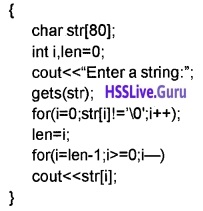### Plus Two Computer Application Arrays Five Mark Questions and Answers

Question 1.
Collect the heights of 12 students from your class in which 7 students are male and others are female students. Suppose these male and female students be seated in two separate benches and you are given a place which is used for sitting these 12 students in linear form. How will you combine and make them sit without mixing male/female students? Write a program for the same.
# include
using namespace std;
int main()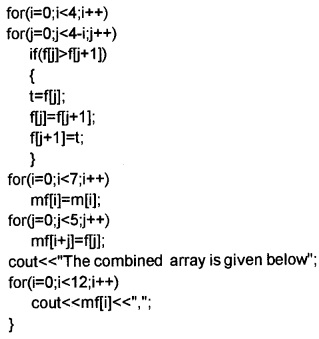Question 2.
Write a program to read 3 marks of 5 students and find the total and display it
# include
using namespace std;
int main()Question 3.
Write a program to read a string and a character and find the character by using linear search.
# include
# include
using namespace std;
int main()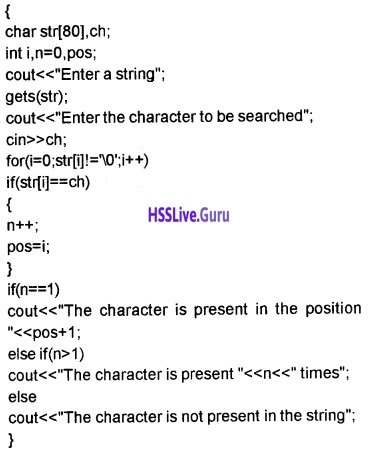Question 4.
Write a program to read a string and find the no. of vowels consonents and special characters.
# include
#include
#include
using namespace std;
int main()Question 5.
Write a program to accept marks of 10 students and find out the largest and smallest mark from the list.

OR

Write a C++ program to store the scores of 10 batsmen of a school cricket team and find the largest and smallest score.
# include
using namespace std;
int main()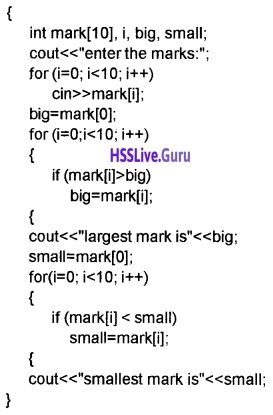Question 6.
Write a C++ program to read 6 marks of a student and find total and average mark.
# include
using namespace std;
int main()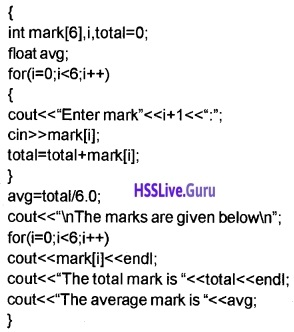Question 7.
Write a C++ proram to accept a sentence and count the number of times the letter ‘s’ occurs in it. For example if the sentence is This is my school’, the output should be 3.